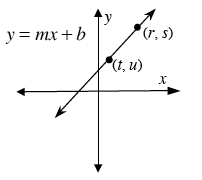### Home > PC > Chapter 7 > Lesson 7.1.1 > Problem7-16

7-16.

Suppose that the point $A$ has coordinates $\left(r, s\right)$, the point $B$ has coordinates $\left(t, u\right)$ and the line $y = mx + b$ goes through both points. Find the distance between $A$ and $B$ in terms of $r$, $t$ and $m$. Hint: First DRAW A PICTURE and then find the slope of the line using the points $A$ and $B$.